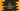# C# how to check for a palindrome number## C# program to check if a number is palindrome or not:

In this post, we will learn how to check if a number is palindrome or not. A number is called palindrome if it is same if we reverse the number. For example, 34543 is a palindrome number.

To check if a number is palindrome or not, we will first reverse that number and compare it with the original number. If both are equal, it is palindrome, else not.

Let’s write down the algorithm first.

### Algorithm to check if a number is palindrome:

1. Get the number as input from the user.
2. Store the value in a different variable. Reverse the number stored in this variable. To reverse it,run one while loop that will pick the rightmost digit of the number and adds it to a final value. Keep adding the rightmost digit and build the reversed number.
3. Compare the original number with the reversed number. If both are equal, it is a palindrome.

### C# program to check for palindrome number:

Below is the complete C# program:

``````using System;
using System.Collections;

public class Program {

static bool isPalindrome(int num) {
int tempValue = num;
int reverse = 0;
while (tempValue > 0) {
reverse = reverse * 10 + tempValue % 10;
tempValue = tempValue / 10;
}

return reverse == num;
}

public static void Main(string[] args) {
int num;
Console.WriteLine("Enter a number :");

if (isPalindrome(num)) {
Console.WriteLine("Entered number is a palindrome number");
} else {
Console.WriteLine("Entered number is not a palindrome number");
}
}
}``````

### Explanation:

1. num in Main method is a variable to hold the user input number. The program is asking the user to enter a number, reading it and storing it in num.
2. isPalindrome method is used to check if a given number is palindrome or not. It takes one number as its argument and returns one boolean value. True if it is a palindrome, False if not.
3. Inside isPalindrome, we are first keeping the value of num in a temporary variable tempValue. We will modify this variable without touching the original num value. i.e. we are reversing the tempValue.
4. To reverse, one while loop is used. This loop runs till the value of tempValue is greater than 0. On each step, it picks the rightmost digit of the number and adds it to the end of reverse. To get the rightmost value, modulo or % is used. Finally, it is changing the value to tempValue/10 , i.e. it removes the rightmost digit from tempValue. At the end of the while loop, reverse will hold the reverse value of tempValue.
5. The return value is reverse == num. i.e. if both num and reverse of num is equal, or if it is a palindrome, it returns True, else False.

### Sample output:

It will give output as like below:

``````Enter a number :
121
Entered number is a palindrome number

Enter a number :
123454321
Entered number is a palindrome number

Enter a number :
1234564321
Entered number is not a palindrome number``````